# The 10-lb-uniform rod AB has a total length of 2L = 2 ft and is attached to collars of negligible mass that slide without friction along fixed rods. If rod AB is released from rest when θ = 30°, determine immediately after release (a) the angular acceleration of the rod, (b) the reaction at A. P16.118

Question-AnswerCategory: Engineering MechanicsThe 10-lb-uniform rod AB has a total length of 2L = 2 ft and is attached to collars of negligible mass that slide without friction along fixed rods. If rod AB is released from rest when θ = 30°, determine immediately after release (a) the angular acceleration of the rod, (b) the reaction at A. P16.118

The 10-lb-uniform rod AB has a total length of 2L = 2 ft and is attached to collars of negligible mass that slide without friction along fixed rods. If rod AB is released from rest when θ = 30°, determine immediately after release (a) the angular acceleration of the rod, (b) the reaction at A.
P16.118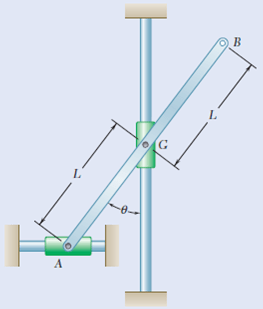Step: 1

Consider the acceleration of the rod AB about its center of gravity.Step: 2

The acceleration of center of gravity G with respect to the point A is given by,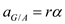Here, r is the distance between point A and G.
Substitute L for r.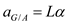From the acceleration triangle, acceleration of G is given by,Substitute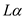for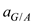.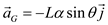Step: 3

Calculate the mass moment of inertia of the rod AB.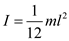Here, m is the mass of the rod and l is the length of the rod.
Substitute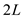for l.Step: 4

Consider the rod AB as the system and model it as a rigid body.
The free body diagram and the mass acceleration diagram of the system are as shown.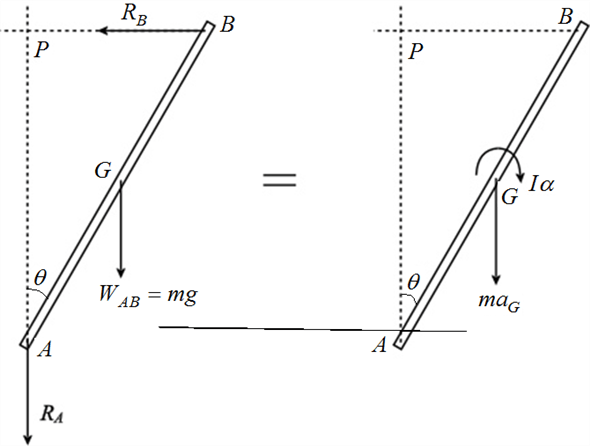Step: 5

(a)
Consider the moment of forces about point P.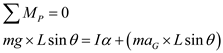Substitute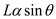forand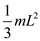forSubstitutefor g,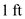for L and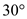for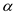.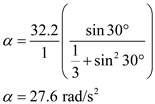Therefore, the angular acceleration of rod AB is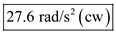.
(b)
Apply force balance in y – direction.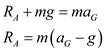Substitutefor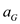.Substitute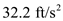for g,for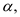1 ft forfor W andfor.Therefore, the reaction at point A is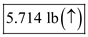.